Maths-
General
Easy

Question

# Find the area of an isosceles triangle whose equal sides are of length 15 cm each and third side is 12 cm.Hint:

## The correct answer is: 18√21 cm2

### It is given that a = 15 cm , b = 15 cm , c = 12 cmUsing Heron’s formula,Area of triangle =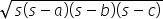where s =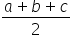s =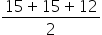= 21Area of triangle =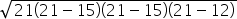=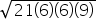=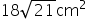So, area of isosceles triangle =NOTE – We can use direct formula of area of isosceles triangle =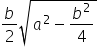where a is an equal side and b is base of trianglea = 15 cm , b = 12 cmArea of isosceles triangle =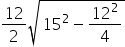=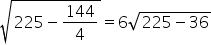= 6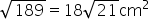#### With Turito Foundation.#### Get an Expert Advice From Turito.